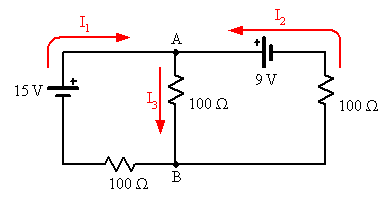# Compute the total power delivered to the circuit by two batteries

## Homework Statement

^^ that is a photo of the circuit

## Homework Equations

P = I*V , but i dont understand how to do it with two loops and two batteries like in this photo.

## The Attempt at a Solution

I have calculated I1 to be .9, I 2 to be .15 and I3 to be .75.

On the very left of the photo is a 15 V battery and the resistor on the bottom that you cant really read is 100 olms.

The other battery (i put a square around the batterys) is 9 V.

Just kinda confused on how to put them together. thnks for your help

gneill
Mentor
What method did you you use to find the currents? Can you show more of your work?
(I ask because they don't look right to me). From what I can tell from your attached picture the circuit looks something like this:#### Attachments

Some suggestion: You allready suggested that there is 2 loops. Define the total potential energy of each loop in terms of the formula V = I*R... So if you choose the first loop, try defining it in terms of the equation. Do the same for the second loop and then you need a third equation seeing that you have 3 variables. How can you define these variables in terms of each other? Not an expert on electrical things but this seems like first principals...

Also when setting your equations, remember that flow goes from positive to negative for your loops, not as you stated in your second loop...

gneill
Mentor
Some suggestion: You allready suggested that there is 2 loops. Define the total potential energy of each loop in terms of the formula V = I*R... So if you choose the first loop, try defining it in terms of the equation.
The power delivered to and consumed by a given circuit can be determined either by summing up all the individual power losses due to resistive elements (sum of P = I2R for each resistor in the circuit), or by multiplying the current leaving the + terminal of each voltage source by the potential of that source (P = I*R) [Edit: Oops, should have been P = I*V there]. and summing the results. Either way, knowledge of the individual currents in the circuit is required, hence the need to write and solve equations based upon Kirchhoff's circuit laws, KVL and KCL.
Do the same for the second loop and then you need a third equation seeing that you have 3 variables. How can you define these variables in terms of each other? Not an expert on electrical things but this seems like first principals...
Actually, two equations suffice to solve for all the currents in a two-loop circuit; since the loops are interacting (they share one or more components), the "third" current is not truly an independent variable.
Also when setting your equations, remember that flow goes from positive to negative for your loops, not as you stated in your second loop...
For purposes of analysis the assumed direction of flow of currents doesn't matter; the mathematics will always sort out the true directions. If the assumed direction for a current turns out to be incorrect, the equations will pop out a negative value for a result. No worries there.

Last edited:
or by multiplying the current leaving the + terminal of each voltage source by the potential of that source (P = I*R) and summing the results.

Actually this is what I ment with flow is from positive to negative, to start at the positive side... I didn't know the actual name of the equation.

Actually, two equations suffice to solve for all the currents in a two-loop circuit; since the loops are interacting (they share one or more components), the "third" current is not truly an independent variable.

With a third equation I ment define I1 in terms of I2 and I3, I guess it is a given just tried to explain it in a understandable manner. Search for unanswered posts and this one came up so thaught I will give it a try seeing that it is frustrating if no help is given.
But thanks for also correcting me, learned something myself...